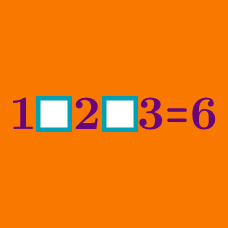Logic

# Multiplication and division only - Operator Search

What series of signs in the three blanks will make the following equation hold true?

$3 \; \square \; 4 \; \square \; 5 \; \square \; 6 = 10$

What series of signs and parentheses on the left side will make the following equality hold true?

$120\; \square \; 2 \; \square \; 3 \; \square \; 4 = 5$

$8 \; \square \; 4 \; \square \; 2 \; \square \; 1$

If we fill in the above blanks using only the operators $\times$ and/or $\div$, is it true that the resultant number is always an integer?

$2 \; \square \; 2 \; \square \; 2 \; \square \; 2$

If we fill in the above blanks using only the operators $\times$ and/or $\div$, which of the following $\color{#D61F06} { \text{cannot} }$ be a resultant number?

What series of signs in the three blanks will $\color{#D61F06} { \text{not} }$ make the following equation true?

$7 \; \square \; 7 \; \square \; 7\; \square \; 7 = 49$

×